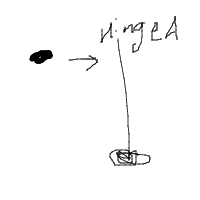# Question about conservation of linear momentum

• RoboNerd
In summary, the ball will collide inelastically with the rod and the rod will start rotating. The angular momentum of the system will be conserved, but linear momentum will not be.f

## Homework Statement[pardon my crude drawing]

Say I have a ball that hits the a rod at rest pivoted around hinge A. The ball in the diagram is the solid black, and the rod is the thin line in the diagram and is rotating around the square-shaped hinge at the bottom. I am not an artist but that is not the point.

The ball will collide inelastically with the rod, and the rod will start rotating. I know angular momentum would be conserved, but would linear momentum be conserved too?

## Homework Equations

conservation of linear momentum. Pi = Pf

## The Attempt at a Solution

Logical reasoning attempts.

[/B]
Thanks so much in advance for the help

The law of conservation of linear momentum states that the total linear momentum of a system will be conserved if the net __________ acting on the system is zero.

Can you fill in the blank?

force

Yes. More specifically, "external force". So, if there is a nonzero external force acting on the system, the linear momentum of the system will not be conserved.

To apply this idea, you need to be clear on what you take to be the system. In particular, what objects are included in the system.

I noticed in your statement of the problem that you mention a hinge A which appears in your drawing to be located at the top of the rod. But you state that the rod rotates about a hinge at the bottom of the rod. I'm a little confused about that. Is there only one hinge?

Yeah sorry, my drawing is a little confusing. Forget the words "hinge a" and assume the hinge is at the bottom of the rod.

I assume my rod and my ball are in a system.

OK. When the ball strikes the rod, do you think the hinge will exert any horizontal force on the end of the rod that is attached to the hinge?

No, it would not. Why would it?

The rod is rotating around, not pushing on the hinge, so the hinge will not exert a reaction force on the rod

Suppose there is no hinge. The rod is at rest in deep space. Imagine the ball hits the rod at its center. The rod would then start moving in translational motion with no rotation. Now imagine that the rod is hinged at its lower end when the ball hits the rod at its center. Instead of the rod moving in pure translation, it now moves in pure rotation about the hinge. So, the hinge must be doing something to the rod during the collision to change the motion from pure translation to pure rotation. Think about the direction of the force of the hinge on the lower end of the rod during the collision.

If the ball hits the rod at some other point than the center of the rod, the hinge will still exert a force on the end of the rod during the collision and the direction of the force will depend on where the ball strikes the rod. There is one exception: If the ball hits the rod at one special point called the "center of percussion", no force will be exerted on the rod by the hinge during the collision. In this special case, linear momentum will be conserved during the collision. For a short video on this concept by a very eccentric man, see

So we have an external hinge force that prevents linear momentum being conserved. Right.

Thanks a lot for the help!